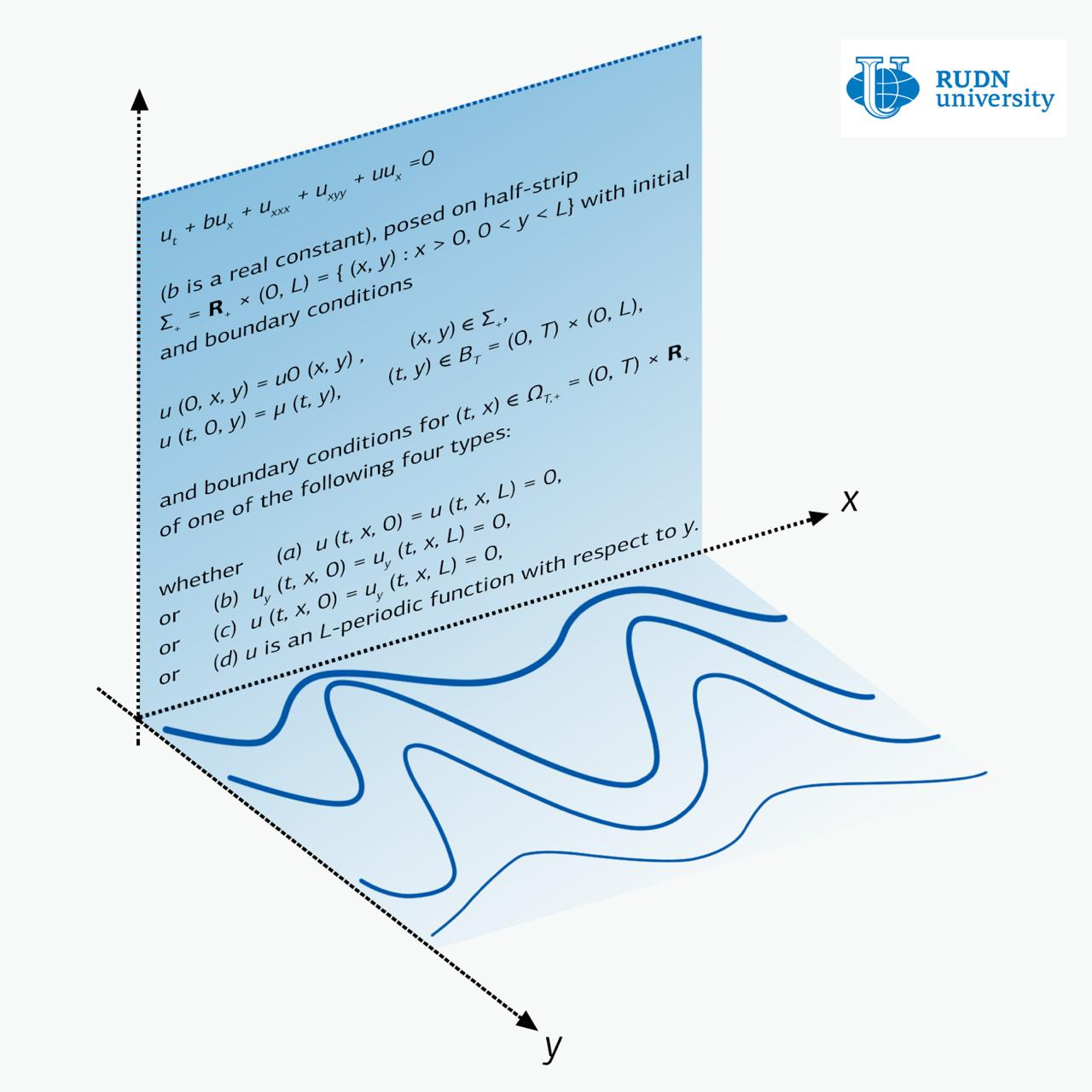RUDN University mathematician first described the movement in a flat strip of plasma

# RUDN University mathematician first described the movement in a flat strip of plasma

RUDN University mathematician for the first time proved the theorem of existence and uniqueness of solutions of the Zakharov-Kuznetsov equation in a strip. Such theorems are very rare for partial differential equations. The new results can be applied, for instance, in astrophysics, in describing the propagation of plane waves in plasma. The article is published in the journal Nonlinear Analysis: Real World Applications.

The Zakharov-Kuznetsov equation is a one-function equation of two variables x and y. For physics, x is the direction of wave propagation, and the deformation of the medium occurs along the perpendicular direction y. For example, an oscillation of the guitar string looks like the wave runs down the string, while the oscillations occur perpendicular direction relative to the run of the wave.

There are a large number of results that describe solutions of the Zakharov-Kuznetsov equations in the case when there are no constraints on y. But the question of wave propagation in the strip — when y is limited — was almost not studied until recently. And this is although such a statement of the problem has a physical meaning, and therefore potential applications.

RUDN University mathematicians dealt with the Zakharov-Kuznetsov equation in the strip. They examined three main cases — when there are no oscillations on the boundary of the strip, when there is no current on the same boundary and when the boundary conditions are periodic in structure. The latter case corresponds to the propagation of waves in a medium whose structure is periodic in x.

In all these cases mathematicians managed to prove theorems of existence and uniqueness of solutions. For systems of partial differential equations, which include the Zakharov-Kuznetsov equation, such equations are very rare.

These results are the first for solutions of the equation with initial conditions in the strip. Flat plasma flows with boundary conditions, which were considered by RUDN University scientists, can occur in physics and astrophysics.

The Zakharov-Kuznetsov equations belong to a wider category of equations known as the Korteweg-de Vries equations. In the study of this category of equations for the first time, it was possible to describe solitons — waves whose shape does not change during movement. Physicists consider solitons as a tool for modern optical data transmission systems. The study of solitons, which can arise in the Zakharov-Kuznetsov equations, is one of the options for the development of the work done by RUDN University mathematicians.News
All news
Science
02 May
The Grand Prix and gold of the Hi-Tech exhibition go to RUDN dentists

April 18-20, the international exhibition of HI-TECH innovations took place in St. Petersburg. Two RUDN University projects received a gold medal and the only Grand Prix at the exhibition.

Science
14 Apr
RUDN Biologists Study Live Microorganisms in Toxic Liquids for Metalworking

RUDN biologists have studied microorganisms that can survive in metalworking fluids. The results will allow “picking up” bacteria and fungi that can process toxic waste fluids into a harmless product.

Science
10 Apr
RUDN University Biologist: salted water saves fish from stress when transporting

RUDN University biologist with colleagues from Brazil and Iran found how to alleviate the stress of fish during transportation. It turned out that this can be achieved with salted water.